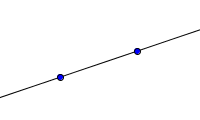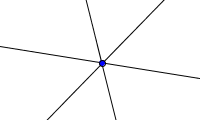﻿ Applying Logical Equivalences to Algebraic and Geometric Statements
APPLYING LOGICAL EQUIVALENCES TO ALGEBRAIC AND GEOMETRIC STATEMENTS

A postulate is a statement that is assumed to be true without proof.
A theorem is a statement that is true, important, and has been proved.

This section presents some basic postulates of geometry and algebra.
You will use these postulates, and other mathematical sentences,
to practice working with logical equivalences.

An English-type sentence like ‘All squares are rectangles’ is actually a mathematical ‘for all..., if... then...’ sentence in disguise.
The word ‘all’ is a very big clue!
You need to make this ‘for all..., if... then...’ structure explicit before working with the sentence mathematically.
The process is illustrated in the next example.

‘For all’ sentences are studied in more detail in a future section, Parallelograms and Negating Sentences.

Consider this true sentence:
(*) All squares are rectangles.
UNIVERSAL SET:
Square and rectangles are both members of the larger population of quadrilaterals.
IMPLICATION FORM of sentence (*):

(**) For all quadrilaterals $\,Q\,$,
if $\,Q\,$ is a square, then $\,Q\,$ is a rectangle.
Use this logical equivalence ... ... to rewrite sentence (**) as ...
 If $\,A\,$ then $\,B\,$ is equivalent to If (not $\,B\,$) then (not $\,A\,$)
For all quadrilaterals $\,Q\,$,
if $\,Q\,$ is not a rectangle, then $\,Q\,$ is not a square.
 If $\,A\,$ then $\,B\,$ is equivalent to (not $\,A\,$) or $\,B\,$
For all quadrilaterals $\,Q\,$,
$\,Q\,$ is not a square, or $\,Q\,$ is a rectangle.

In the sentence ‘Two distinct points determine a line’,
you don't see the word ‘all’ to clue you that it's a ‘for all..’ sentence in disguise.
This sentence is explored in the next example.

Geometry Postulate:
(*) Two distinct points determine a line.
Note: distinct means different
UNIVERSAL SET:
the set of all points
IMPLICATION FORM of sentence (*):

(**) For all points $\,P\,$ and $\,Q\,$,
if $\,P\,$ and $\,Q\,$ are distinct, then $\,P\,$ and $\,Q\,$ determine a unique line.
THE IDEA:Use this logical equivalence ... ... to rewrite sentence (**) as ...
 If $\,A\,$ then $\,B\,$ is equivalent to If (not $\,B\,$) then (not $\,A\,$)
For all points $\,P\,$ and $\,Q\,$,
if $\,P\,$ and $\,Q\,$ do not determine a unique line,
then $\,P\,$ and $\,Q\,$ are not distinct.
 If $\,A\,$ then $\,B\,$ is equivalent to (not $\,A\,$) or $\,B\,$
For all points $\,P\,$ and $\,Q\,$,
$P\,$ and $\,Q\,$ are not distinct,
or $\,P\,$ and $\,Q\,$ determine a unique line.

The next example uses a familiar result from algebra.
Again, it's a ‘for all...’ sentence in disguise.

the ZERO FACTOR LAW
(*) If two numbers multiply to zero, then at least one of the numbers is zero.
UNIVERSAL SET:
the set of all real numbers
MATHEMATICAL FORM of sentence (*):

(**) For all real numbers $\,a\,$ and $\,b\,$,
if $\,ab=0\,$ then $\,((a=0)\text{ or }(b=0))\,$
THE IDEA:
I'm thinking of two numbers that multiply to zero.
Can you tell me anything about the numbers that I'm thinking of?
Yes! At least one of them must equal zero.
Use these logical equivalences ... ... to rewrite sentence (**) as ...
 If $\,A\,$ then $\,B\,$ is equivalent to If (not $\,B\,$) then (not $\,A\,$)

 not($\,A\,$ or $\,B\,$) is equivalent to (not $\,A\,$) and (not $\,B\,$)
For all real numbers $\,a\,$ and $\,b\,$,
if $\,a\ne 0\,$ and $\,b\ne 0\,$,
then $\,ab\ne 0\,$.
 If $\,A\,$ then $\,B\,$ is equivalent to (not $\,A\,$) or $\,B\,$

 ($\,A\,$ or $\,B\,$) or $\,C\,$ is equivalent to $\,A\,$ or ($\,B\,$ or $\,C\,$)
For all real numbers $\,a\,$ and $\,b\,$,
$\,ab\ne 0\,$ or $\,a=0\,$ or $\,b=0\,$.

Note that the associativity of ‘or’
allowed us to drop all parentheses in this rephrasing.

The table below summarizes more results that you should know:

Geometry Postulate:
Three noncollinear points determine a unique plane.Substitution
If $\,a=b\,$, then $\,a\,$ and $\,b\,$ can be substituted,
one for the other, in any expression.
Numbers have lots of different names!
Use whatever name is most convenient in a given situation.

For all real numbers $\,a\,$, $\,b\,$, and $\,c\,$: $$a=b\ \ \iff\ \ a+c=b+c$$
This result gives you something that you can do to an equation
that will always yield an equivalent equation.

The Addition Property of Equality says that you can
add (or subtract) the same number to (or from) both sides of an equation,
and it will not change the truth of the equation.
Multiplication Property of Equality

For all real numbers $\,a\,$ and $\,b\,$, and for $\,c\ne 0\,$: $$a=b \ \ \iff\ \ ac=bc$$
This result gives you something that you can do to an equation that will always yield an equivalent equation.

The Multiplication Property of Equality says that you can
multiply (or divide) both sides of an equation by the same nonzero number,
and it will not change the truth of the equation.

Notice that multiplying both sides of an equation by zero
can change the truth of the equation:
‘$\,3=5\,$’   is false, but   ‘$\,3\cdot 0=5\cdot 0\,$’   is true.
Negating Equations and Inequalities:

 STATEMENT NEGATION $a=b$ $a\ne b$ $a\lt b$ $a\ge b$ $a\gt b$ $a\le b$
You must be able to negate each of these sentences in the specified form.
Negating an Implication:

 not($\,A\Rightarrow B\,$) is equivalent to $\,A\,$ and (not $\,B\,$)
An implication is false when the hypothesis is true and the conclusion is false.

Finally, never lose sight of the following key facts!

Two sentences are equivalent if they always have the same truth values:
if one is true, so is the other; if one is false, so is the other.

Sentences that are equivalent can be used interchangeably, and a mathematician will use whichever is easiest.

Equivalence is such an important mathematical concept that there are many ways to say the same thing.
The following four sentences are completely interchangeable: $$\begin{gather} A\text{ is equivalent to }B\cr A\text{ if and only if }B\cr A\text{ iff }B\cr A\iff B \end{gather}$$

Master the ideas from this section